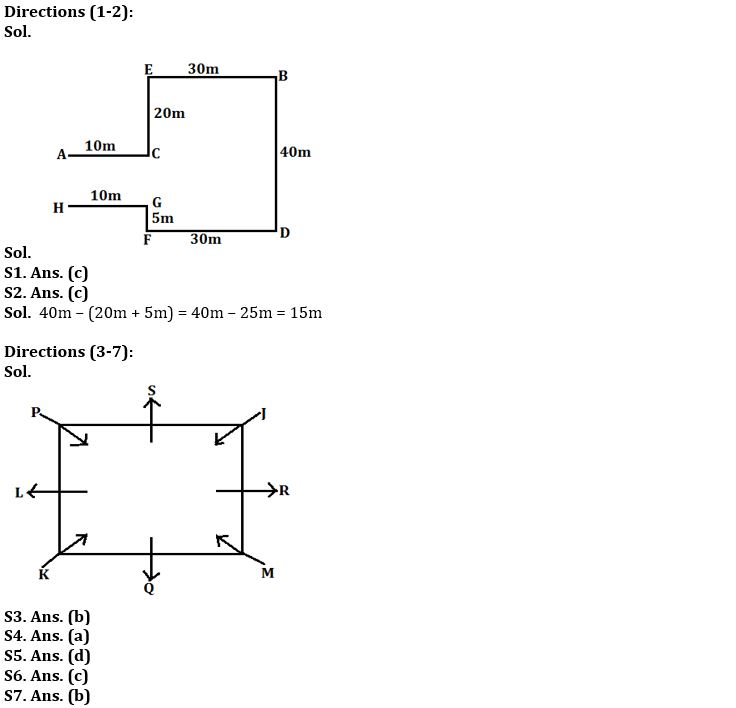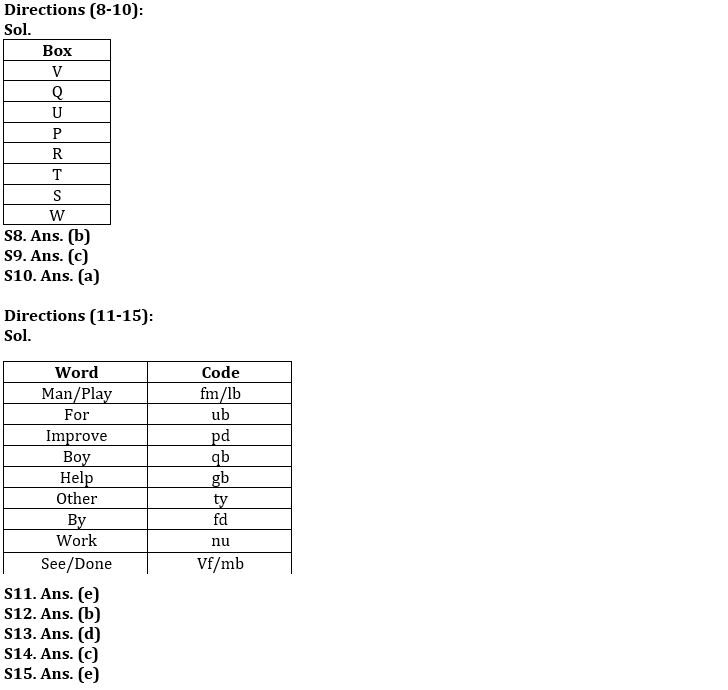Latest Banking jobs   »

# Reasoning Ability Quiz For Bank Foundation 2022- 24th December

Directions (1-2): Study the following information carefully and answer the questions given below.
Point A is 10m West of point C. Point C is 20m South of point E. Point E is 30m West of point B. Point D is 40m South of point B. Point F is 5m South of point G. Point H is 10m West of point G. Point F is 30m West of point D.

Q1. Point H is in which direction from point A?
(a) West
(b) South west
(c) South
(d) North east
(e) South east

Q2. What is the shortest distance between Point C to Point G?
(a) 10m
(b) 20m
(c) 15m
(d) Can^’ t be determine
(e) None of these

Directions (3-7): Study the following information carefully and answer the questions given below:
Eight persons J, K, L, M, P, Q, R and S are the sitting around the square table in such a way four of them sit at four corners and face the center while four of them sit in the middle of each of the four sides and face outside.
J faces the center and sits third to the left of Q. P faces the center and is not an immediate neighbour of Q. Only one person sits between Q and R. M sits second to right of K, who faces the center. L is not an immediate neighbour of J.

Q3. Who among the following are immediate neighbours of S?
(a) R, M
(b) P, J
(c) P, L
(d) J, M
(e) K, M

Q4. What is the position of L with respect to P?
(a) Immediate right
(b) Second to the right
(c) Immediate left
(d) Third to the left
(e) None of these

Q5. Four of the following five belong to a group in a certain way, find who among the following does not belong to the group?
(a) S
(b) L
(c) R
(d) M
(e) Q

Q6. Who among the following person sits opposite to R?
(a) S
(b) M
(c) L
(d) K
(e) None of these

Q7. How many maximum persons can sit between P and M?
(a) 6
(b) 3
(c) 2
(d) 4
(e) 5

Directions (8-10): Study the following information carefully and answer the given questions.
Eight boxes P, Q, R, S, T, U, V and W are placed one above the another, but not necessarily in the same order. Two boxes are placed between U and T. U is placed above T. One box is placed between U and V. Three boxes are placed between P and W. P is placed immediately below U. Two boxes are placed between R and W. Q is placed above S.

Q8. Which of the following box is placed at top?
(a) Q
(b) V
(c) U
(d) W
(e) None of these

Q9. How many boxes are placed between Box T and Box W?
(a) None
(b) Two
(c) One
(d) Four
(e) Three

Q10. Which of the following box is placed at bottom?
(a) W
(b) R
(c) T
(d) S
(e) None of these

Directions (11-15): Study the following information and answer the following questions:
In a certain code language-
‘Man play for improve’ is written as ‘fm lb pd ub’,
‘Boy for help other’ is written as ‘ub ty qb gb’,
‘Improve other by work’ is written as ‘fd pd nu ty’,
‘Boy see work done’ is written as ‘qb nu vf mb’,

Q11. What is the code for ‘see’ in the given code language?
(a) qb
(b) vf
(c) nu
(d) mb
(e) Cannot determined

Q12. Which among the following word is written as ‘gb’ in the given code language?
(a) work
(b) help
(c) man
(d) boy
(e) None of these

Q13. Which among the following word is written as ‘fd pd’ in the given code language?
(a) play improve
(b) man done
(c) by work
(d) improve by
(e) None of these

Q14.What is the possible code for ‘other time’ in the given code language?
(a) wc fd
(b) mb vf
(c) ty wc
(d) rb mb
(e) ty ub

Q15. Which among the following word is written as ‘fm’ in the given code language?
(a) man
(b) boy
(c) done
(d) play
(e) Either (a) or (d)

Solutions#### Congratulations!Union Budget 2023-24: Free PDF